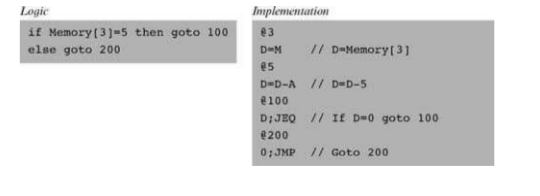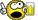# A bit confused on the pointer notationClassicListThreaded7 messagesOpen this post in threaded view
|

## A bit confused on the pointer notation

 Please refer to time stamp 3:05 of Unit 1.4's video. Pseudo Assembly Code: D = *p Statements made in the video: 1. *p refers to the (value stored at) memory location that p points at. 2.  If we said instead D = p, then presumably we'll get the value 257 because that's what p points to. 3.  p is equivalent to 0. 4.  D = p will leave us the contents of ram. My thoughts and concerns on the above statements: 1.  I am okay with this. It's just de-referencing a pointer. p is a pointer and if I de-reference it, I am accessing the contents of that address. This is my knowledge from C++. In C++, we can de-reference as many times as we want. Ex: int num = **p This first retrieves the value(address) stored at address p, and then retrieves the values at that address. 2,3.  Now I am getting confused. If p is an address, say 0, then shouldn't D = p leave D = 0?         Is there a implicit de-reference occurring here?       4.  An implicit de-reference seems to be what is occurring then.      D = p in C would actually be D = *p      and      D = *p in C would actually be D = **p. Am I misunderstanding this? Thanks your time. https://youtu.be/Iq9KA6qRqXo?t=181
Open this post in threaded view
|

## Re: A bit confused on the pointer notation

 Administrator By "Unit 1.4" are you referring to the Coursea course? If so, I don't have access to it. Since nothing in the Hack assembly, VM, or Jack languages use pointers, let alone define the notation and semantics associated with that notation, there's no basis for knowing what/when/where implicit dereferencing might be happening. IIRC, the authors got a bit sloppy when describing the call and return command using their pointer-like pseudocode and had some inconsistencies.
Open this post in threaded view
|

## Re: A bit confused on the pointer notation

 This post was updated on . I posted the link to the video at the very bottom(I don't think the embeds are working correctly). I think the videos are from the coursea. I see. They translated this: D = *p to this Hack code: @p A = M D = M I guess I will try not to worry about it too much. Thanks for your time.
Open this post in threaded view
|

## Re: A bit confused on the pointer notation

 In reply to this post by WBahn quoting 4.3.1 Now, when the compiler later encounters references to array cells like foo[j], it translates them as follows. First, note that the jth array entry should be physically located in a memory location that is at a displacement j from the array’s base address (assuming, for simplicity, that each array element uses a single word). Hence the address corresponding to the expression foo[j] can be easily calculated by adding the value of j to the value of foo. Thus in the C programming language, for example, a command like x=foo[j] can be also expressed as x=*(foo+j), where the notation “*n” stands for “the value of Memory[n]”. When translated into machine language, such commands typically generate the following code (depending on the assembly language syntax): Looks a bit pointery.. I had a look at the example that cyclogenesis posted D = *p to this Hack code: @p A = M D = M which on first sight I thought were equivalent, but now I'm not sure,  M is the content held at the address at  the @ value and then my mind went. I can only describe it as occasionally I write down a word and it doesn't look like it's spelt correctly (even though it is). The more it write it out the more it looks like gobble-de-gook.  So I was tottally confident that the two bit of code were equivalent, now having done some research I'm not so sure.. Sorry I've been tottally unhelpful, appologies to you both !
Open this post in threaded view
|

## Re: A bit confused on the pointer notation

 This is from the book. Taking the first couple of lines in this exampleD = *p to this Hack code: @p A = M D = M I think D=*p should be @p D=MPlease correct me if I'm wrong !...